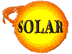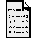What would happen if we transplanted some Sun-matter to the middle of San Francisco?This answer is courtesy of Tom Duvall, GSFC/Stanford Solar Oscillations Investigations

The question is how a person in Menlo Park (60 km away from San Francisco) might be affected by placing a cubic centimeter of matter from the center of the sun on top of the Transamerica Building in San Francisco.

The short answer is that the person would get a dose of x-ray radiation that is small compared to the normal exposure of humans in a year to natural sources of radiation. How I came to this conclusion is discussed below.

To answer this question, we need two constants from a model of the sun. These are the mass density at the center of the sun, rhoc = 160 g/cm³, and the temperature at sun center, Tc = 15 * 106 °K. So the mass of our cubic centimeter of material is 160g. From thermodynamics, we know that each particle of this material has an average thermal energy of kTc, where k=8.62 * 10-5 eV/° is Boltzmann's constant. By multiplying this out, we get kTc=1290 eV approximately equal to 1.3 keV. A photon with this amount of energy is in the x-ray region of the spectrum. So I will assume that in the resultant explosion, the thermal energy in the cubic centimeter of material is all converted to x-rays. This is not exactly what will happen but will give the worst-case scenario for radiation damage.

To get the total amount of thermal energy, we need to multiply the thermal energy per particle, kTc by the number of particles in our 160 g of material. If we make the assumption that the material is ionized hydrogen gas (not completely true but not too far from reality), we can get the number of protons by dividing by the mass of the proton, mp=1.67*10-24 g to get 9.6*1025 protons. Then, with one electron for each proton, we double this number to get the total number of particles N=19.2*1025. We then multiply by the thermal energy per particle to get the total energy 4 * 1017 ergs, where I have substituted the more convenient energy unit, 1 erg = 6.25* 1011 eV.

This amount of energy is sent out from the explosion.

A person at some distance from the explosion will intercept a certain fraction of the radiation which will depend on the distance from the explosion. To get the fraction of the energy intercepted, we will divide the cross-sectional area of a person by the area of the sphere of radius the distance from San Francisco to Menlo Park, say 60 km. For a 6 ft person of average width 26 cm, we get a cross section of 4.8*10³ cm². The area of the sphere is 4* pi*r², or 4.5*1014 cm². So, by multiplying the fraction by the total energy, we get that the person intercepts 4*106 ergs of energy.

To assess the damage of radiation to living things, the important quantity is the amount of energy absorbed per gram of mass of the living thing. Assuming a 150 lb person ( 6.8*104 g), we get 58 ergs/g of energy absorbed. Using the approximate conversion factor of 1 rem = 100 ergs/g, (see http://xxx.lanl.gov/abs/astro-ph/9505028) we get 0.6 rem for the dosage. From this same reference we find that this is quite a bit less than our normal dose of 5 rem/year that we get from natural sources.

Back to the FAQsBack to the SOLAR CenterComments?

Special Thanks to T. Duvall.